PointsBet 2019 Stanley Cup Odds
+550
5.5 to 1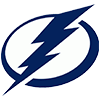Lightning
11.9% implied probability

+750
7.5 to 1Bruins
9.1% implied probability

+850
8.5 to 1Avalanche
8.1% implied probability

+900
9 to 1Penguins
7.7% implied probability

+950
9.5 to 1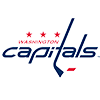Capitals
7.3% implied probability

+1000
10 to 1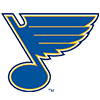Blues
7.0% implied probability

+1300
13 to 1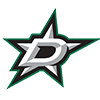Stars
5.5% implied probability

+1400
14 to 1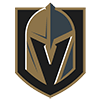Golden Knights
5.1% implied probability

+2000
20 to 1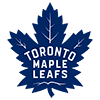Maple Leafs
3.7% implied probability

+2000
20 to 1Oilers
3.7% implied probability

+2000
20 to 1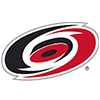Hurricanes
3.7% implied probability

+2200
22 to 1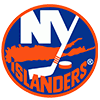Islanders
3.4% implied probability

+2500
25 to 1Canucks
3.0% implied probability

+3000
30 to 1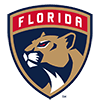Panthers
2.5% implied probability

+3000
30 to 1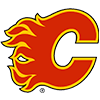Flames
2.5% implied probability

+3000
30 to 1Predators
2.5% implied probability

+3000
30 to 1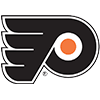Flyers
2.5% implied probability

+3500
35 to 1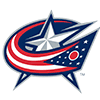Blue Jackets
2.1% implied probability

+3500
35 to 1Coyotes
2.1% implied probability

+5000
50 to 1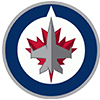Jets
1.5% implied probability

+6000
60 to 1Wild
1.3% implied probability

+8000
80 to 1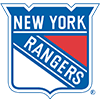Rangers
1.0% implied probability

+9000
90 to 1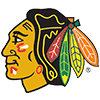Blackhawks
0.8% implied probability

+12500
125 to 1Canadiens
0.6% implied probability

+15000
150 to 1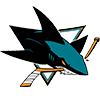Sharks
0.5% implied probability

+15000
150 to 1Sabres
0.5% implied probability

+30000
300 to 1Ducks
0.3% implied probability

+100000
1000 to 1Senators
0.1% implied probability

+100000
1000 to 1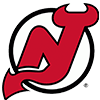Devils
0.1% implied probability

+100000
1000 to 1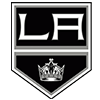Kings
0.1% implied probability

+250000
2500 to 1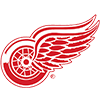Red Wings
0.0% implied probability